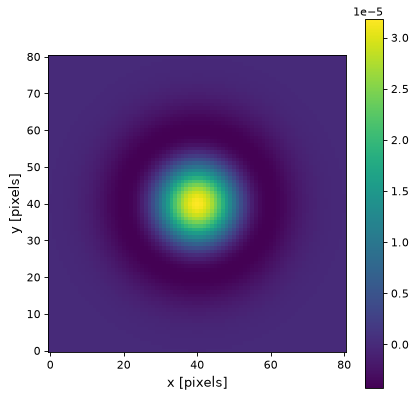# RickerWavelet2DKernel¶

class `astropy.convolution.``RickerWavelet2DKernel`(width, **kwargs)[source]

2D Ricker wavelet filter kernel (sometimes known as a “Mexican Hat” kernel).

The Ricker wavelet, or inverted Gaussian-Laplace filter, is a bandpass filter. It smooths the data and removes slowly varying or constant structures (e.g. Background). It is useful for peak or multi-scale detection.

This kernel is derived from a normalized Gaussian function, by computing the second derivative. This results in an amplitude at the kernels center of 1. / (pi * width ** 4). The normalization is the same as for `scipy.ndimage.gaussian_laplace`, except for a minus sign.

Note

See https://github.com/astropy/astropy/pull/9445 for discussions related to renaming of this kernel.

Parameters
widthnumber

Width of the filter kernel, defined as the standard deviation of the Gaussian function from which it is derived.

x_size`int`, optional

Size in x direction of the kernel array. Default = ⌊8*width +1⌋.

y_size`int`, optional

Size in y direction of the kernel array. Default = ⌊8*width +1⌋.

mode`str`, optional
One of the following discretization modes:
• ‘center’ (default)

Discretize model by taking the value at the center of the bin.

• ‘linear_interp’

Discretize model by performing a bilinear interpolation between the values at the corners of the bin.

• ‘oversample’

Discretize model by taking the average on an oversampled grid.

• ‘integrate’

Discretize model by integrating the model over the bin.

factornumber, optional

Factor of oversampling. Default factor = 10.

Examples

Kernel response:

```import matplotlib.pyplot as plt
from astropy.convolution import RickerWavelet2DKernel
ricker_2d_kernel = RickerWavelet2DKernel(10)
plt.imshow(ricker_2d_kernel, interpolation='none', origin='lower')
plt.xlabel('x [pixels]')
plt.ylabel('y [pixels]')
plt.colorbar()
plt.show()
```

(png, svg, pdf)